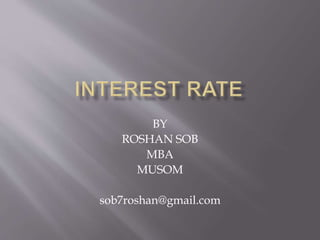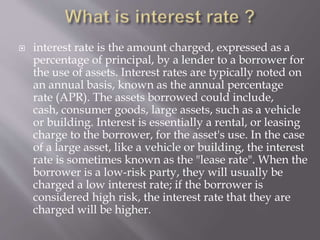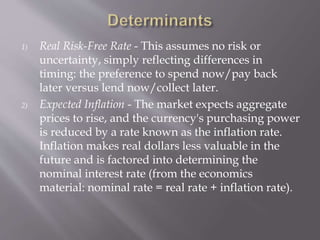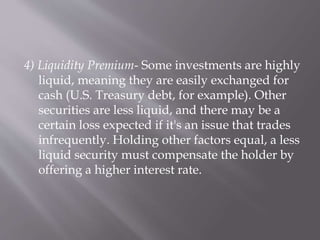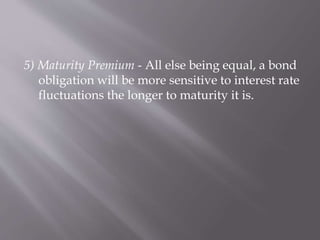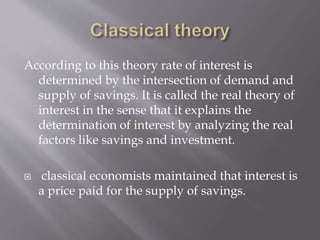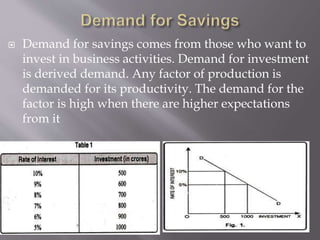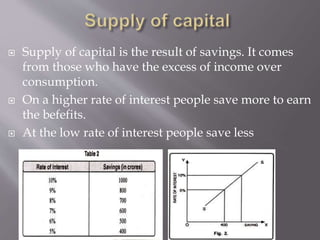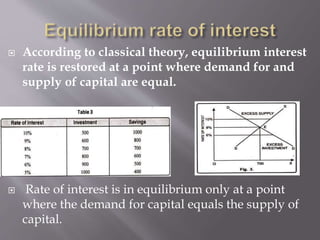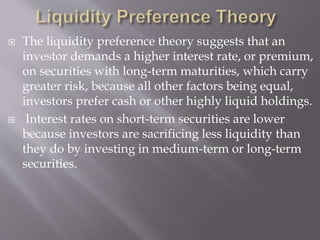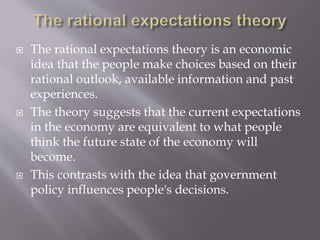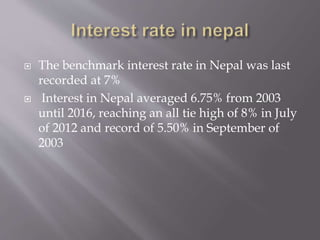1 de 13

### Interest rate

1. BY ROSHAN SOB MBA MUSOM sob7roshan@gmail.com
2.  interest rate is the amount charged, expressed as a percentage of principal, by a lender to a borrower for the use of assets. Interest rates are typically noted on an annual basis, known as the annual percentage rate (APR). The assets borrowed could include, cash, consumer goods, large assets, such as a vehicle or building. Interest is essentially a rental, or leasing charge to the borrower, for the asset's use. In the case of a large asset, like a vehicle or building, the interest rate is sometimes known as the "lease rate". When the borrower is a low-risk party, they will usually be charged a low interest rate; if the borrower is considered high risk, the interest rate that they are charged will be higher.
3. 1) Real Risk-Free Rate - This assumes no risk or uncertainty, simply reflecting differences in timing: the preference to spend now/pay back later versus lend now/collect later. 2) Expected Inflation - The market expects aggregate prices to rise, and the currency's purchasing power is reduced by a rate known as the inflation rate. Inflation makes real dollars less valuable in the future and is factored into determining the nominal interest rate (from the economics material: nominal rate = real rate + inflation rate).
4. 3) Default-Risk Premium - What is the chance that the borrower won't make payments on time, or will be unable to pay what is owed? This component will be high or low depending on the creditworthiness of the person or entity involved.
5. 4) Liquidity Premium- Some investments are highly liquid, meaning they are easily exchanged for cash (U.S. Treasury debt, for example). Other securities are less liquid, and there may be a certain loss expected if it's an issue that trades infrequently. Holding other factors equal, a less liquid security must compensate the holder by offering a higher interest rate.
6. 5) Maturity Premium - All else being equal, a bond obligation will be more sensitive to interest rate fluctuations the longer to maturity it is.
7. According to this theory rate of interest is determined by the intersection of demand and supply of savings. It is called the real theory of interest in the sense that it explains the determination of interest by analyzing the real factors like savings and investment.  classical economists maintained that interest is a price paid for the supply of savings.
8.  Demand for savings comes from those who want to invest in business activities. Demand for investment is derived demand. Any factor of production is demanded for its productivity. The demand for the factor is high when there are higher expectations from it
9.  Supply of capital is the result of savings. It comes from those who have the excess of income over consumption.  On a higher rate of interest people save more to earn the befefits.  At the low rate of interest people save less
10.  According to classical theory, equilibrium interest rate is restored at a point where demand for and supply of capital are equal.  Rate of interest is in equilibrium only at a point where the demand for capital equals the supply of capital.
11.  The liquidity preference theory suggests that an investor demands a higher interest rate, or premium, on securities with long-term maturities, which carry greater risk, because all other factors being equal, investors prefer cash or other highly liquid holdings.  Interest rates on short-term securities are lower because investors are sacrificing less liquidity than they do by investing in medium-term or long-term securities.
12.  The rational expectations theory is an economic idea that the people make choices based on their rational outlook, available information and past experiences.  The theory suggests that the current expectations in the economy are equivalent to what people think the future state of the economy will become.  This contrasts with the idea that government policy influences people's decisions.
13.  The benchmark interest rate in Nepal was last recorded at 7%  Interest in Nepal averaged 6.75% from 2003 until 2016, reaching an all tie high of 8% in July of 2012 and record of 5.50% in September of 2003